## Solutions of Reflection of Light Lakhmir Singh Manjit Kaur VSAQ, SAQ, MCQ, and HOTS, and Pg No. 199 Class 10 Physics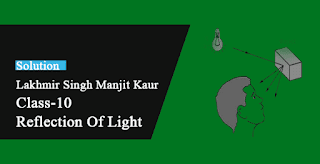18. An object 3 cm high is placed at a distance of 10 cm in front of a converging mirror of focal length 20 cm.
Find the position, nature and size of the image formed.

→ U = 10 cm
F = 20 cm
Height of object = 3 cm
Such that using the mirror formula, we get
v = +20 cm;
The image formed is behind the converging mirror; Virtual and erect ; 6 cm high

19. A concave mirror has a focal length of 4 cm and an object 2 cm tall is placed 9 cm away from it. Find the nature, position and size of the image formed.

→ Focal length = 4cm
Height of object = 2cm
Object distance from mirror = 9 cm
Such that after using mirror formula we get image distance , v =-7.2 cm
the nature, position and size of the image formed is real and inverted ; at a distance of 7.2 cm in front of concave mirror and 1.6 cm high.

20. When an object is placed 20 cm from a concave mirror, a real image magnified four times is formed. Find:
(a) the focal length of the mirror.
(b) Where must the object be placed to give a virtual image three times the height of the object?

→ Given:-
u =-20 cm, m=- 4, for the real image21. A dentist's mirror has a radius of curvature of 3 cm. How far must it be placed from a small dental cavity to give a virtual image of the cavity that is magnified six times ?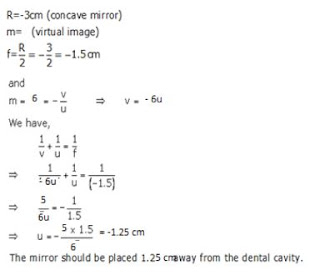22. A large concave mirror has a focus length of 0.75 cm. A person stands 10 m in front of the mirror. Where is the person's image ?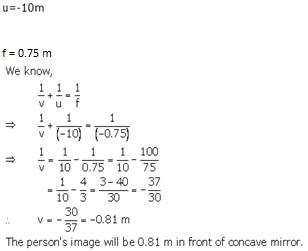0.81 m in front of the concave mirror.

23. An object of 5.0 cm size is placed at a distance of 20.0 cm from a converging mirror of radius of curvature 30.0 cm. At what distance from the mirror should a screen be placed to get the sharp image ? Also calculate the size of the image.

→ Radius of curvature = 30.0 cm
F = R/2 = 30/2 = 15 cmThe screen should be placed 60 cm in front of the mirror.
And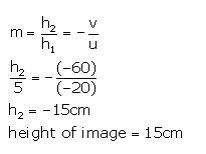24. A concave mirror produces three times enlarged virtual image of an object placed at 10 cm in front of it. Calculate the focal length of the mirror.

→ m=3 (virtual image)
u=-10cm
R=?
We know thatFocal length of the mirror is 15 cm.

25. A bright object 100 mm high stands on the axis of a concave mirror of focal length 100 mm and at a distance of 300 mm from the concave mirror. How big will the image be ?

→ h1=100 mm, f=-100 mm, u=-300 mm,  h2=?
We have:26. How far should an object be placed from the pole of a converging mirror of radius of curvature 40 cm to form a real image of the size exactly 4th the size of the object?

→ Radius of curvature = 40 cm
F = R/2 = 40/2 = 20 cm
f= -20 cm, m= -1/4 (real image)
We know that27. When an object is placed at a distance of 50 cm from a concave spherical mirror, the magnification produced is, - Where should the object be placed to get a magnification of, -1/8?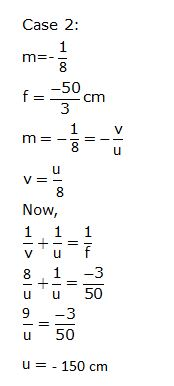28. An object is placed (a) 20 cm, (b) 4 cm, in front of a concave mirror of focal length 12 cm. Find the nature and position of the image formed in each case.

→ (a) The image distance from the pole is -30 cm; the nature and position of the image formed in each case is that image is formed at a distance of 30 cm in front of mirror (on its left side); Real and inverted
(b) The image distance from the pole is + 6 cm; the nature and position of the image formed in each case is that image is formed at a distance of 6 cm behind the mirror (on its right side); Virtual and erect.

29. A concave mirror produces a real image 1 cm tall of an object 2.5 mm tall placed 5 cm from the mirror. Find the position of the image and the radius of curvature of the mirror.

→  h2=1cm=10mm (real image), h1=2.5mm, u = -5cm= -50 mm.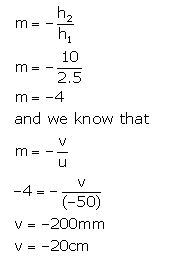The image is formed 20 cm in front of the mirror.
And,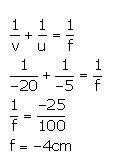Radius of curvature = 2f = -8 cm.

30. A man holds a spherical shaving mirror of radius of curvature 60 cm at a distance of 15 cm, from his nose. Find the position of image, and calculate the magnification.

→ Radius of curvature, R= -60cm
F = R/2 = 60/2 = 30cm
f= -30cm, u= -15cm
We have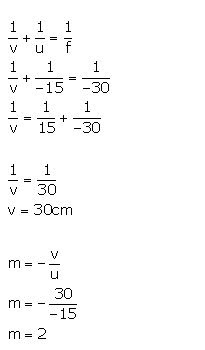So, the image is formed 30 cm behind the mirror and the magnification is +2.

31A. An object is placed just outside the principal focus of concave mirror. Draw a ray diagram to show how the image is formed, and describe its size, position and nature.The image is formed beyond the centre of curvature of the mirror and the nature of the image is real, inverted and magnified.

31B. If the object is moved further away from the mirror, what changes are there in the position and size of the image ?

→ If the object is moved further away from the mirror, the image is formed nearer to the mirror and its size goes on decreasing.31C. An object is 24 cm away from a concave mirror and its image is 16 cm from the mirror. Find the focus and radius of curvature of the mirror.

→ u= -24cm
v= -16cm
R=?, m=?
we know that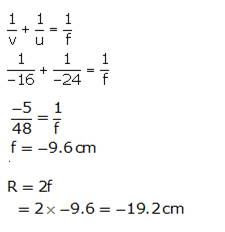Multiple Choice Questions (MCQs)-Pg-199

32. Linear magnification produced by a concave mirror may be :
A. m≤1
B. m≥1
C. 1<m≥1
D. 1<m>1

→ Magnification, m = v/u.

33. Magnification produced by a convex mirror is always :
A. m> 1
B. m<1
C. m = 1
D. 1> m<1

→ Magnification, m = v/u.

34. Magnification produced by a plane mirror is:
A. m>1
B. m<1
C. m = 0
D. m = 1

→ Magnification, m = v/u
m= 1/1 = 1.

35. In order to obtain a magnification of, -3 (minus 3) with a concave mirror, the object should be placed:
A. between pole and focus
B. between focus and centre of curvature
C. at the centre of curvature
D. beyond the centre of curvature

→ Magnification, m = v/u.

36. A concave mirror produces a magnification of + 3. The object is placed:
A. at the focus
B. between focus and centre of curvature
C. between focus and pole
D. beyond the centre of curvature

→ Magnification, m = v/u.

37. If a magnification of, -2.5 (minus two point one) is to be obtained by using a converging mirror, then the object has to be placed:
A. between pole and focus
B. at the centre of curvature
C. beyond the centre of curvature
D. at infinity

→ Magnification, m = v/u.

38. In order to obtain a magnification of,- 0.9 (minus 0.9) with a concave mirror, the object must be placed:
A. at the focus
B. between pole and focus
C. between focus and centre of curvature
D. beyond the centre of curvature

→ Magnification, m = v/u.

39. An object is placed at a large distance in front of a concave mirror of radius of curvature 60 cm. The image will be formed in front of the mirror at a distance of :
A. 20 cm
B. 30 cm
C. 40 cm
D. 50 cm

→ R = 60 cm.
f = -30 cm.

40. In order to obtain a magnification of, -1.5 with a concave mirror of focal length 20 cm, the object will have to be placed at a distance :
A. between 6cm and 20 cm
B. between 40 cm and 20 cm
C. between 48 cm and 40 cm
D. beyond 64 cm

→ m = -1.5 = v/u
such that v = -1.5u
f = -20 cm
using mirror formula, find u.

41. Linear magnification (m) produced by a rear view mirror fitted in vehicles:
A. m = 1
B. m >1
C. m <1
D. 1<m>1

→ Magnification, m = v/u.

Questions Based on High Order Thinking Skills (HOTS)-Pg-200

42. Between which two points of concave mirror should an object be placed to obtain a magnification of :
(a) - 3 (b) + 2.5 (c) - 0.4

→ (a) mirror should an object be placed Between focus and centre of curvature to obtain a magnification of -3
(b) mirror should an object be placed Between pole and focus to obtain a magnification of 2.5

(c) mirror should an object be placed Beyond the centre of curvature to obtain a magnification of -0.4

43. At what distance from a concave mirror of focal length 20 cm should an object be placed so that:
(a) its real image is formed 20 cm from the mirror?
(b) its virtual image is formed 20 cm from the mirror?44. If a concave mirror has a focal length of 20 cm, find the two positions where an object can be placed to give, in each case, an image twice the height of the object.45. A mirror forms an image which is 30 cm from an object and thrice its height.
(a) Where must the mirror be situated?
(b) What is the radius of curvature?
(c) Is the mirror convex or concave?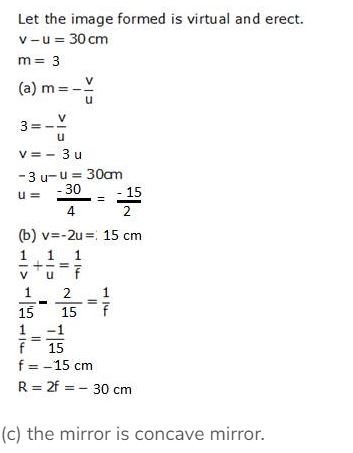1. What type of image/images are formed by:
(a) a convex mirror?
(b) a concave mirror?

→ (a) Virtual and erect image is formed by a convex mirror.

(b) Virtual and erect; Real and inverted images are formed by a concave mirror.

2. Which mirror has a wider field of view ?

→ Convex mirror.

3. If you want to see an enlarged image of your face, state whether you will use a concave mirror or a convex mirror?

→ Concave mirror will be used to see an enlarged image of our face.

4. Which mirror always produces a virtual, erect and diminished image of an object ?

→ Convex mirror.

5. An object is placed at a long distance in front of a convex mirror of radius of curvature 38cm. State the position of its image.

→ The position of its image is At focus ; 19 cm behind convex mirror.

6. Name the spherical mirror which can produce a real and diminished image of an object.

→ Concave mirror can produce a real and diminished image of an object.

7. Name the spherical mirror which can produce a virtual and diminished image of an object.

→ Convex mirror can produce a virtual and diminished image of an object.

8. One wants to see a magnified image of an object in a mirror. What type of mirror should one use?

→ Concave mirror should be used to get a magnified image of an object in a mirror.

9. Name the mirror which can give:
(a) an erect and enlarged image of an object.
(b) an erect and diminished image of an object.

→ (a) Concave mirror can give an erect and enlarged image of an object.
(b) Convex mirror can give an erect and diminished image of an object.

10. State whether the following statement is true or false: A converging mirror is not used as a rear-view mirror.

→ True

11. What type of mirror could be used:
(a) as a shaving mirror?
(b) as a shop security mirror?

→ (a) Concave mirror could be used as a shaving mirror.
(b) Convex mirror could be used as a shop security mirror.

12. Which type of mirror is usually used as a rear-view mirror in motor cars ?

→ Convex mirror is usually used as a rear-view mirror in motor cars.

13. What kind of mirrors are used in shopping centres to watch the activities of the customers?

→ Convex mirrors are used in shopping centres to watch the activities of the customers.

14. A ray of light going towards the of a convex mirror becomes parallel to the principal axis after reflection from the mirror. Draw a labelled diagram to represent this situation.15. Fill in the following blank with a suitable word:
A ray of light which is parallel to the' principal axis of a _______, appears to be coming from focus after reflection from the mirror.

→ Convex mirror.

16. Why does a driver prefer to use a convex mirror as a rear-view mirror in a vehicle ?

→ A driver prefers to use a convex mirror as a rear-view mirror because convex mirror produces an erect image of the objects as well as has wider field of view.

17. Why can you not use a concave mirror as a rear-view mirror in vehicles?

→ We cannot use a concave mirror as a rear-view mirror in vehicles as concave mirror produces inverted images due to which all the vehicles will be seen running upside down in the mirror.

18. Where would the image be formed by a convex mirror if the object is placed:
(a) between infinity and pole of the mirror?
(b) at infinity ?
Draw labelled ray-diagrams to show the formation of image in both the cases.

→ (a) Image will form between pole and focus by a convex mirror if the object is placed by a convex mirror if the object is placed
(b) Image will form At focus by a convex mirror if the object is placed by a convex mirror if the object is placed.

19. The shiny outer surface of a hollow sphere of aluminium of radius 60cm is to be used as a mirror :
(a) What will be the focal length of this mirror?
(b) Which type of spherical mirror will it provide ?
(c) State whether this spherical mirror will diverge or converge light rays.

→ (a) R=60cm
f=?
We know that
f=R/2 = 60/2 = 30 cm
(b) It will provide convex mirror
(c) diverge light rays.

20. What is the advantage of using a convex mirror as a rear-view mirror in vehicles as compared to a plane mirror? Illustrate your answer with the help of labelled diagrams.

→ The advantage of using a convex mirror as a rear-view mirror in vehicles as compared to a plane mirror is that a convex mirror has a wider field of view as compared to a plane mirror. which enables driver to view much larger area of the traffic behind him.21. Give two uses of a convex mirror. Explain why you would choose convex mirror for these uses.

→ Two uses of convex mirror:
1) Convex mirrors are used inside buildings:
Large hospitals, offices or stores sometimes make use of convex mirrors in order to let people see what is around a corner to avoid people running into each other and prevent minor/major collisions.
2) Convex mirrors are used in vehicles:
They are used as a rear view mirror in vehicles because the mirror can diverge a beam of light and makes a virtual image. And as the focal length and radius of curvature of the convex mirror are virtual the image is always produced up the right way and that too smaller in size than the actual size of the object. So the mirror is able to give a wide view of the field.

22. What would your image look like if you stood close to a large :
(a) convex mirror?
(b) concave mirror ?

→ (a) Our image will be diminished, virtual and erect if we stand close to a large convex mirror because when the object lies anywhere between the pole and inifinity, the concave mirror forms a diminished, virtual and erect image.
(b) image will be enlarged, virtual and erect if we stand close to a large concave mirror because when the object lies within the focus of a concave mirror, it forms an enlarged, virtual and erect image.

23. Which of the following are concave mirrors and which convex mirrors ? Shaving mirrors, Car headlight mirror, Searchlight mirror, Driving mirror, Dentist's inspection mirror, Torch mirror, Staircase mirror in a double- decker bus, Make-up mirror, Solar furnace mirror, Satellite TV dish, Shop security mirror.24. How will you distinguish between a plane mirror, a concave mirror and a convex mirror without touching them?

→ We can distinguish between a plane mirror, a concave mirror and a convex mirror by bringing our face close to each mirror, turn by turn.25. If a driver has one convex and one plane rear-view mirror, how would the images in each mirror appear different?The images formed in the convex rear-view mirror will be smaller than those formed in the plane rear-view mirror.

26A. Draw a labelled ray diagram to show the formation of image of an object by a convex mirror. Mark clearly the pole, focus and centre of curvature on the diagram.26B. What happens to the image when the object is moved away from the mirror gradually?

→ The size of the image goes on decreasing.

26C. State three characteristics of the image formed by a convex mirror.

→ Virtual, erect and diminished.

27A. Draw a labelled ray diagram to show the formation of image in a convex mirror when the object is at infinity. Mark clearly the pole and focus of the mirror in the diagram.27B. State three characteristics of the image formed in this case.

→ Nature of image is virtual, erect, diminshed.

27C. Draw diagram to show how a convex mirror can be used to give a large field of view.Multiple Choice Questions (MCQs)-Pg-206

28. The image formed by a spherical mirror is virtual. The mirror will be
A. concave
B. convex
C. concave/convex
D. metallic

→ Image formed can be virtual in both the mirror cases that is concave as well as convex.

29. Whatever be the position of the object, the image formed by a mirror is virtual, erect and smaller than the object. The mirror then must be :
A. concave
B. convex
C. plane
D. metallic

→ Image formed can be virtual in both the mirror cases that is concave as well as convex.

30. The mirror used by a dentist to examine the teeth of a person is :
A. concave
B. convex
C. plane
D. metallic

→ Concave mirror will be used to see an enlarged image of our teeth.

31. If the image formed is always virtual, the mirror can be :
A. concave or plane
B. convex or plane
C. concave/convex
D. plane

→ Image formed by plane mirror and convex mirror is always virtual.

32. A concave mirror cannot be used as :
A. a dentist's mirror
B. a torch reflector
C. a magnifying mirror
D. arear view mirror

→ Convex mirror is used as a rear view mirror.

33. A boy is standing in front of and close to a special mirror. He finds the image of his head bigger than normal, the middle part of his body of the same size, and his legs smaller than normal. The special mirror is made up of three types of mirrors in the following order from top downwards :
A. Concave, Plane, Convex
B. Plane, Convex, Concave
C. Convex, Plane, Concave
D. Convex, Concave, Plane34. The mirror which can form a magnified image of an object is :
A. concave
B. convex
C. plane
D. metallic

→ Concave mirror will be used to see an enlarged image.

35. A real image of an object is to be obtained. The mirror required for this purpose is :
A. concave
B. convex
C. plane
D. metallic

→ Concave and plane always gives virtual.

36. Consider two statements A and B given below :
A : real image is always inverted
B : virtual image is always erect
Out of these two statements :
A. only A is true
B. only B is true
C. both A and B are true
D. Neither A and B is true

→ Plane mirror and convex mirror gives virtual and erect image.

Questions Based on High Order Thinking Skills (HOTS)-Pg-207

37. The diagrams show the appearance of crayons when placed in front of and close to two mirrors A and B, turn by turn.(a) Which mirror is convex?
(b) Which mirror is concave ? Give reasons for your choice.

→ (a) Mirror B is convex ; It forms a smaller image of crayon.

(b) Mirror A is concave ; It forms a larger image of crayon.

38. The diagram shows a dish antenna which is used to receive television signals from a satellite. The antenna (signal detector) is fixed in front of the curved dish.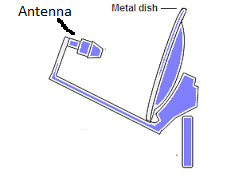(a) What is the purpose of the dish?
(b) Should it be concave or convex ?
(c) Where should the antenna be positioned to receive the strongest possible signals ?
(d) Explain what change you would expect in the signals if a larger dish was used.

→ (a) the purpose of the dish is To collect a large amount of TV signals from the satellite
(b) It should be Concave mirror.
(c) the antenna be positioned to receive the strongest possible signals At the focus of the dish.
(d) If larger dish was used stronger signals will be received.

39. A man standing in front of a special mirror finds his image having a very small head, a fat body and legs of normal size. What is the shape of :
(a) top part of the mirror?
(b) middle part of the mirror ?
(c) bottom part of the mirror? Give reasons for your choice.

→ (a) the shape of top part of the mirror is Convex mirror as it forms smaller image
(b) the shape of middle part of the mirror is Concave mirror as it forms bigger image
(c) the shape of bottom part of the mirror is Plane mirror as it forms image of same size.

40. Two big mirrors A and B are fitted side by side on a wall. A man is standing at such a distance from the wall that he can see the erect image of his face in both the mirrors. When the man starts walking towards the mirrors, he finds that the size of his face in mirror A goes on decreasing but that in mirror B remains the same.
(a) mirror A is concave and mirror B is convex
(b) mirror A is plane and mirror B is concave
(c) mirror A is concave and mirror B is plane
(d) mirror A is convex and mirror B is plane

→ (d) Mirror A is convex and mirror B is plane.

1. An object is kept at a distance of 5 cm in front of a convex mirror of focal length 10 cm. Calculate the position and magnification of the image and state its nature.

→ Object distance = 5cm
Focal length = 10 cm
Image distance after using the formula = 3.3 cm
Magnification = v/u = 3.3/5 = 0.66

Nature of the image is virtual and erect.

2. An object is placed at a distance of 10 cm from a convex mirror of focal length 5 cm.
(i) Draw a ray-diagram showing the formation of image.
(ii) State two characteristics of the image formed.
(iii) Calculate the distance of the image from mirror.

→ Object distance = 10cm
Focal length = 5 cm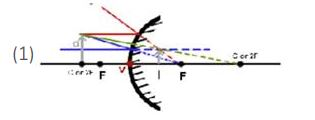(ii) The image formed is Virtual and erect as well as Diminished.
(iii) Using the mirror formula,
Image formation = 3.3 cm behind the convex mirror.

3. An object is placed at a distance of 6 cm from a convex mirror of focal length 12 cm. Find the position and nature of the image.

→ Object distance = 6 cm
Focal length = 12 cm
Uisng mirror formula,
Image distance = 4 cm behind the mirror
The natue of the image is Virtual and erect
Magnification , m = v/u = 4/6 = 0.66 ,
Hence the image formed is diminished.

4. An object placed 20 cm in front of a mirror is found to have an image 15 cm
(a) in front of it,
(b) behind the mirror.
Find the focal length of the mirror and the kind of mirror in each case.

→ Object distance, u = -20cm
(a) Image distance = -15 cm
Using the mirror formula, we get
focal length=  cm
The kind of mirror is concave mirror.
(b) Image distance = 15cm
Using the mirror formula, we get
focal length = 60 c-m
The kind of mirror is convex mirror.

5. An arrow 2.5cm high is placed at a distance of 25 cm from a diverging mirror of focal length 20 cm. Find the nature, position and size of the image formed.

→ Height of object = 2.5cm
Object distance from mirror = -25cm
Focal length = -20cm
Using the mirror formula,
Image distance, v = 11.1 cm
Thus, the image is formed 11.1 cm behind the convex mirror and is Virtual and erect.
Magnification, m = v/u = 1.1 cm tall.

6. A convex mirror used as a rear-view mirror in a car has a radius of curvature of 3 m. If a bus is located at a distance of 5 m from this mirror, find the position of image. What is the nature of the image ?

→ Radius of curvature , R = 3m
F = R/2 = 3/2 = 1.5 m
Object distance from mirror = (-5m)= u( As it is on left of the mirror)
Using the mirror formula,Putting the Values in above equation we get:-
Image distance = 1.15 m behind the mirror;
The nature of the image formed is Virtual and erect.

7. A diverging mirror of radius of curvature 40 cm forms an image which is half the height of the object. Find the object and image positions.

→ Radius of curvature , R = 40 cm
F = R/2 = 40/2 = 20 cm
Magnification, m = 1/2
v/u = 1/2
such that u = 2v
Using the mirror formula,
Object distance = 20cm
And the image distance = 10cm
behind the mirror.

8. The radius of curvature of a convex mirror used as a rear view mirror in a moving car is 2.0 m. A truck is coming from behind it at a distance of 3.5 m. Calculate
(a) position, and
(b) size, of the image relative to the size of the truck. What will be the nature of the image ?

→ Radius of curvature , R = 2m
F = R/2 = 2/2 = 1 m
Object distance from mirror = 3.5m
Using the mirror formula,
(a) Image distance is 0.77 m behind the mirror
(b)m = v/u = 4.5 ; The nature of the image is Virtual and erect.

9A. Draw a diagram to represent a convex mirror. On this diagram mark principal axis, principal focus F and the centre of curvature C if the focal length of convex mirror is 3 cm.B. An object 1 cm tall is placed 30 cm in front of a convex mirror of focal length 20 cm. Find the size and position of the image formed by the convex mirror.

→ Focal length = 3cm
Height of object = 1cm
Object distance = -20cm
Using mirror formula,
Image distance = 0.4 cm and size of the image is 12 cm behind the mirror.

Questions Based on High Order Thinking Skills (HOTS)-Pg-209

10. A shop security mirror 5.0 m from certain items displayed in the shop produces one-tenth magnification.
(a) What is the type of mirror ?
(b) What is the radius of curvature of the mirror ?

→ (a) the type of mirror is Convex mirror
(b) Magnification = 1/10 = v/u
u = 10v
Object distance = 5m
Using mirror formula,
Focal length = 4.5m

11. An object is placed 15 cm from
(a) a converging mirror, and
(b) a diverging mirror, of radius of curvature 20 m. Calculate the image position and magnification in each case.

→ (a) Object distance = 15cm
F = R/2 = 20/2 = 10m
Using mirror formula for concave lens,
Image distance, v = -30 cm
The image is formed 30 cm in front of converging mirror;
Magnification, m = v/0u = -2
(b) Object distance = 15cm
F = R/2 = 20/2 = 10m
Using mirror formula for convex lens,
Image distance v = + 6 cm
The image is formed 6 cm behind the diverging mirror ;
Magnification, m = v/u =0.4.

12. An object 20cm from a spherical mirror gives rise to a virtual image 15 cm behind the mirror. Determine the magnification of the image and the type of mirror used.